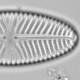## Statigraphic diagrams with ggplot

`rioja::strat.plot` is a great tool for plotting stratigraphic plots in R, but sometimes it is not obvious how to do something I want, perhaps a summary panel showing the percent trees/shrubs/herbs. Of course, I could extend `strat.plot`, but I do all nearly all my figures with `ggplot` now and wouldn’t know where to start.

In this post, I’m going to show how to make a stratigraphic plot with `ggplot`.

First I’m going to download the pollen data for Tsuolbmajavri (Seppä and Hicks, 2006) from Neotoma.

```library("neotoma")
library("tidyverse")

#get dataset list
pollen_sites <- get_dataset(datasettype = "pollen", x = 15733)

pollen_data <- pollen_data[]

#species groups to plot
wanted <- c("TRSH", "UPHE", "VACR", "SUCC", "PALM", "MANG")
```

Now I need to extract and combine the pollen count, the chronology and the taxon information from the downloaded neotoma data. I `gather` the data to make it into a long thin (tidy) table, filter the taxa that are in the ecological groups I want to plot and calculate percentages.

```thin_pollen <- bind_cols(
counts(pollen_data),
ages(pollen_data) %>% select(depth, age, age.type)
) %>%
gather(key = taxon, value = count, -depth, -age, -age.type) %>%
left_join(pollen_data\$taxon.list, c("taxon" = "taxon.name")) %>%
filter(ecological.group %in% wanted) %>%
group_by(depth) %>%
mutate(percent = count / sum(count) * 100)
```

Now I can plot the data after a little further processing: I’m selecting taxa that occur at least three times and with a maximum abundance of at least 3%, and making taxon into a factor so taxa from the same ecological group will plot together.

I use `geom_area` twice, once to show the 10x the percent, once to show the percent. I then need to use `ylim` in `coord_flip` to truncate the axes at the maximum pollen abundance. The plot uses facets to show the taxa separately.

```thin_pollen %>%
mutate(taxon = factor(taxon, levels = unique(taxon[order(ecological.group)]))) %>%
group_by(taxon) %>%
filter(max(percent) >= 3, sum(percent > 0) >= 3) %>%
ggplot(aes(x = age, y = percent, fill = ecological.group)) +
geom_area(aes(y = percent * 10), alpha = 0.3, show.legend = FALSE) +
geom_area(show.legend = FALSE) +
coord_flip(ylim = c(0, max(thin_pollen\$percent))) +
scale_x_reverse(expand = c(0.02, 0)) +
scale_y_continuous(breaks = scales::pretty_breaks(n = 2)) +
facet_wrap(~ taxon, nrow = 1) +
labs(x = expression(Age~''^{14}*C~BP), y = "Percent", fill = "Group") +
theme(strip.text.x = element_text(angle = 90, size = 7, hjust = 0),
panel.spacing = unit(x = 1, units = "pt"),
axis.text.x = element_text(size = 7)
)
```I don’t think that is too bad for a first attempt, but I think I’ll stick to `rioja::strat.plot` most of the time.1.Melissa Sehrt says: